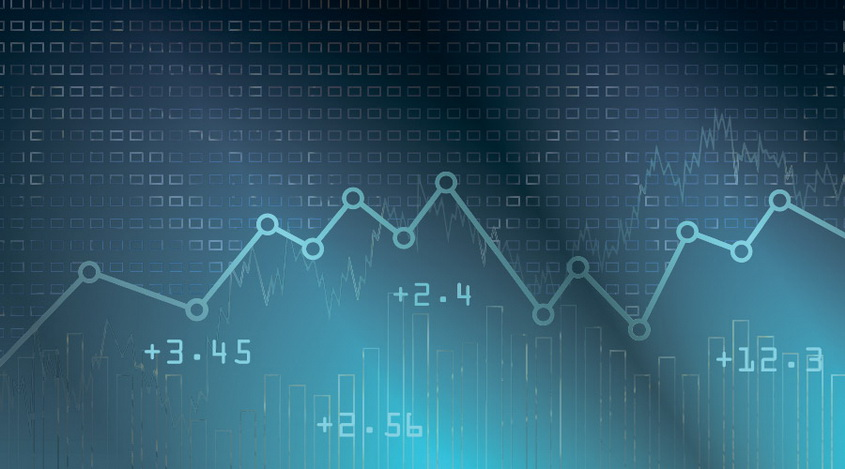# Smoothing Moving Averages

Monday, 28 August 2017 16:32Moving averages are a standard tool for technical analysis of the Forex market, which displays the average values of the asset for a certain period. This tool is installed by default in the MetaTrader4 terminal.

When calculating the simple moving average, all the prices have the same value. However, trading on the Forex, the recent price values are often much more important for a trader then the previous ones. For such a reason, other types of lines appeared, such as smoothed, exponentially smoothed, linear weighted.

In the MetaTrader4 terminal, the line type can be selected in the “MA Method” drop-down menu:

• Simple. A simple MA, in the construction of which all the values are equally significant;
• Exponential. When calculating this line, the last candle is most important. This method suits better for trading the short positions;
• Smoothed. The smoothed curve is suitable for opening long positions. Indications of this line change only with the significant fluctuations;
• Linear Weighted. The recent value of the asset is more important.

## Choosing MA Method

The difference between the MA types can be traced by changing the timeframe. More values of the price are taken into account for the calculations with longer periods. Therefore, this type of instrument becomes more sensitive.

Let the period be equal to 15 candles. Then, during the curve calculation, prices from the first to the fifteenth candle will be taken into account. As soon as the new bar is drawn, the formula will consider candles with numbers from 2 to 16. When calculating the smoothed line, the 16th candle will be the most significant one, in the case of a simple one - all the prices will be considered equally.

Smoothing is used to react more quickly to the price fluctuations.

The formula for calculating a simple MA based on three points is as follows:.

Here is the formula for a weighted MA, also for three points:.

You cannot single out one type of moving average, which would be ideal for any trader. The method of MA depends on the strategy used and on the time frames as well.

### Smoothing Moving Average Method

Here is the algorithm of calculations.

1. Calculations are based on the time range of n elements: y1, y2, ..., yn. The smoothing interval will be equal to m (m <n). If it’s necessary to smooth out the minor fluctuations, a longer range is more useful. If small changes are needed, the interval can be reduced. Experts recommend the unique range values.
2. The arithmetical mean of the first m levels of the y1, y2, ..., yn series is calculated. It will be the smoothed value of the central sections of the interval. Next, the range is shifted to the right side by one level. Then the calculation of the arithmetic mean is repeated.

The formula: n-m+1 determines the number of final values of the series level.

It’s worth noting that the first and the last levels will not be smoothed.

The described type of tools is widely used in technical analysis. The signal to open the positions can be the intersection of lines with different periods. It happens when the trend changes. The faster line goes towards the slower one, and then crosses it:It should be noted that the flat periods on the market cause a lot of false signals, which requires the use of additional tools to filter the readings.

It’s also possible to use a single line. In this case, the trade is made at the intersection with the price levels. When the line crosses the level from top to bottom, it’s a good time for opening the sale order. Otherwise, it’s time to buy.